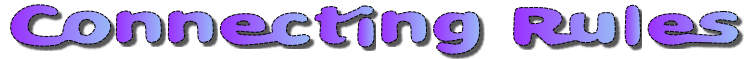#x = 3

y = 4

## A Mathematics Lesson Starter Of The Day

Topics: Starter | Algebra

• S Curtis, Brune Park CC
•
• One of my more industrious students thought it clever to sequence it as follows:
y-1 = x
2y-5 = x
3y-9 = x etc...
• Heeley's Y7 brainboxes, Rawthorpe High, Huddersfield
•
• We thought this was bare cushtie (this means very good)! we recognised that there are an infinite number of possibilities.
Watch out for Mr Heeley on Teacher's TV on May 5th at 10pm. (Sky channel 880, Virgin 240).
God bless you all at Transum
Love Y7 xx
• N. Cox, Woodbridge, Suffolk
•
• Excellent open-ended starter which led to work with powers, brackets roots and many different types of equations.
• Jules, Longton High School
•
• 5. More like this please!
• 7ab/M4 The Best Maths Class In KA's, King Alfred's College OXON
•
• Some students in the class came up with these examples:
yxyxxyxxxxy=524288
yxyyx=2048
Then we thought about squaring the values.
• Luke, Savio Salesian College
•
• My class were very good at this starter,i think you should make a similar one.
• Transum,
•
• This starter provides an open ended opportunity for pupils to demonstrate what they can remember about algebra. It is a suitable activity for most ability levels and a quick walk around the classroom to see what pupils are writing provides a quick assessment of their understanding and imagination.
• Mr Anderson, Garth Hill College
•
• This is a good warm up for any class.

How did you use this starter? Can you suggest how teachers could present or develop this resource? Do you have any comments? It is always useful to receive feedback and helps make this free resource even more useful for Maths teachers anywhere in the world.

Previous Day | This starter is for 20 April | Next Day

There are of course no end of correct answers and here are some examples.

There is at least one mistake. Can you find the errors when x=3 and y=4?

 $$x + y = 7$$ $$x - y = -1$$ $$y - x = 1$$ $$2x + 3y = 18$$ $$5x - 2y = 12$$ $$4y - x = 13$$ $$xy - 10 = 9$$ $$180 - 2x + y = 178$$ $$20x - 3xy = 24$$ $$x^2 + y = 13$$ $$10x - y^2 = 14$$ $$y^2 - x^2 = 7$$ $$x^2y + 13 = 49$$ $$x^2y^2 - 2x = 138$$ $$x^2y^2 = 576$$ $$x! + y = 10$$ $$5(x + y) = 52$$ $$3y \div x = 4$$ $$\frac{xy}{2}= 25$$ $$\frac{84}{xy}= 27$$ $$\frac{15y}{5x}= y$$

Note to teacher: Doing this activity once with a class helps students develop strategies. It is only when they do this activity a second time that they will have the opportunity to practise those strategies. That is when the learning is consolidated. Click the button above to regenerate another version of this starter from random numbers.

Your access to the majority of the Transum resources continues to be free but you can help support the continued growth of the website by doing your Amazon shopping using the links on this page. Below is an Amazon link. As an Amazon Associate I earn a small amount from qualifying purchases which helps pay for the upkeep of this website.

Educational Technology on AmazonTeacher, do your students have access to computers such as tablets, iPads or Laptops?  This page was really designed for projection on a whiteboard but if you really want the students to have access to it here is a concise URL for a version of this page without the comments: Transum.org/go/?Start=April20 However it would be better to assign one of the student interactive activities below.Here is the URL which will take them to a related student activity.

Transum.org/go/?to=Connect